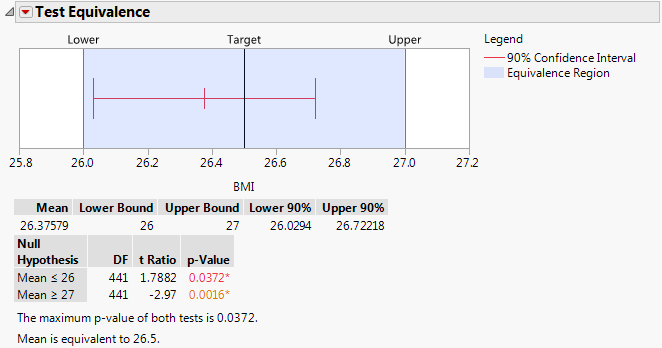Publication date: 11/29/2021

## Test Equivalence

Equivalence tests assess whether a population mean is equivalent to a hypothesized value. You must define a threshold difference that is considered equivalent to no difference. The Test Equivalence option uses the Two One-Sided Tests (TOST) approach. Two one-sided t tests are constructed for the null hypotheses that the difference between the true mean and the hypothesized value exceeds the threshold. If both null hypotheses are rejected, this implies that the true difference does not exceed the threshold. You conclude that the mean can be considered practically equivalent to the hypothesized value.

When you select the Test Equivalence option, you specify the Hypothesized Mean, the threshold difference (Difference Considered Practically Zero), and the Confidence Level. The Confidence Level is 1 - alpha, where alpha is the significance level for each one-sided test.

The Test Equivalence report in Figure 3.11 is for the variable BMI in the Diabetes.jmp sample data table. The Hypothesized Mean is 26.5 and the Difference Considered Practically Zero is specified as 0.5.

Figure 3.11 Test Equivalence ReportThe report shows the following:

A plot of your defined equivalence region that shows the Target and boundaries, defined by vertical lines labeled Lower and Upper.

A confidence interval for the calculated mean. This confidence interval is a 1 - 2*alpha level interval.

A table that shows the calculated mean, the specified lower and upper bounds, and a (1 - 2*alpha) level confidence interval for the mean.

A table that shows the results of the two one-sided tests.

A note that summarizes the results, and states whether the mean can be considered equivalent to the Target value.